# PostgreSQL Tutorial: Using CAST To Convert a Value of One Type to Another

August 2, 2023

Summary: in this tutorial, we will show you how to use PostgreSQL `CAST` operator to convert a value of one type to another.

## Introduction to PostgreSQL `CAST` operator

There are many cases that you want to convert a value of one data type into another. PostgreSQL provides you with the `CAST` operator that allows you to do this.

The following illustrates the syntax of type `CAST`:

``````CAST ( expression AS target_type );
``````

In this syntax:

• First, specify an expression that can be a constant, a table column, an expression that evaluates to a value.
• Then, specify the target data type to which you want to convert the result of the expression.

### PostgreSQL type cast :: operator

Besides the type `CAST` syntax, you can use the following syntax to convert a value of one type into another:

``````expression::type
``````

See the following example:

``````SELECT
'100'::INTEGER,
'01-OCT-2015'::DATE;
``````

Notice that the cast syntax with the cast operator (::) is PostgreSQL-specific and does not conform to the SQL standard

## PostgreSQL `CAST` examples

Let’s take some examples of using the `CAST` operator to convert a value of one type to another.

### 1) Cast a string to an integer example

The following statement converts a string constant to an integer:

``````SELECT
CAST ('100' AS INTEGER);
``````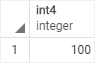If the expression cannot be converted to the target type, PostgreSQL will raise an error. See the following example:

``````SELECT
CAST ('10C' AS INTEGER);
``````
``````ERROR:  invalid input syntax for integer: "10C"
LINE 2:  CAST ('10C' AS INTEGER);
``````

### 2) Cast a string to a date example

This example uses the `CAST` to convert a string to a date:

``````SELECT
CAST ('2015-01-01' AS DATE),
CAST ('01-OCT-2015' AS DATE);
``````First, we converted `2015-01-01` literal string into `January 1st 2015`. Second, we converted `01-OCT-2015` to `October 1st 2015`.

### 3) Cast a string to a double example

In the following example, we try to convert a string `'10.2'` into a double value:

``````SELECT
CAST ('10.2' AS DOUBLE);
``````

Whoops, we got the following error message:

``````ERROR:  type "double" does not exist
LINE 2:  CAST ('10.2' AS DOUBLE)
``````

To fix this, you need to use `DOUBLE PRECISION` instead of `DOUBLE` as follows:

``````SELECT
CAST ('10.2' AS DOUBLE PRECISION);
``````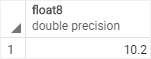### 4) Cast a string to a boolean example

This example uses the `CAST()` to convert the string ‘true’, ‘T’ to true and ‘false’, ‘F’ to false:

``````SELECT
CAST('true' AS BOOLEAN),
CAST('false' as BOOLEAN),
CAST('T' as BOOLEAN),
CAST('F' as BOOLEAN);
``````

Here is the output: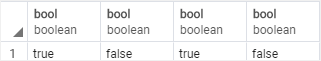### 5) Convert a string to a timestamp example

This example uses the cast operator (::) to convert a string to a timestamp:

``````SELECT '2019-06-15 14:30:20'::timestamp;
``````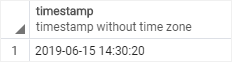### 6) Convert a string to an interval example

This example uses the cast operator to convert a string to an interval:

``````SELECT '15 minute'::interval,
'2 hour'::interval,
'1 day'::interval,
'2 week'::interval,
'3 month'::interval;
``````

Here is the output: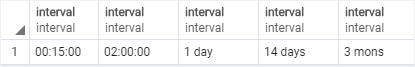### 7) Using `CAST` with table data example

First, create a `ratings` table that consists of two columns: id and rating. The data type of the `rating` column is `VARCHAR(1)`:

``````CREATE TABLE ratings (
ID serial PRIMARY KEY,
rating VARCHAR (1) NOT NULL
);
``````

Second, insert some sample data into the `ratings` table.

``````INSERT INTO ratings (rating)
VALUES
('A'),
('B'),
('C');
``````

Because the requirements change, we use the same `ratings` table to store ratings as number e.g., 1, 2, 3 instead of A, B, and C:

``````INSERT INTO ratings (rating)
VALUES
(1),
(2),
(3);
``````

So the `ratings` table stores mixed values including numeric and string.

``````SELECT
*
FROM
ratings;
``````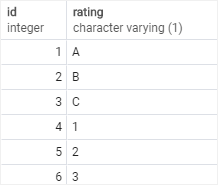Now, we have to convert all values in the `rating` column into integers, all other A, B, C ratings will be displayed as zero. To do this, you use the `CASE` expression with the type `CAST` as shown in the following query:

``````SELECT
id,
CASE
WHEN rating~E'^\\d+\$' THEN
CAST (rating AS INTEGER)
ELSE
0
END as rating
FROM
ratings;
``````

The `CASE` checks the rating, if it matches the integer pattern, it converts the rating into an integer, otherwise, it returns 0.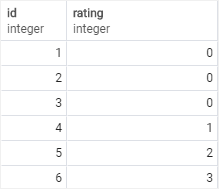In this tutorial, you have learned how to use PostgreSQL `CAST` to convert a value of one type to another.